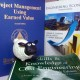# W16 – ABM- Follow up on Learning Curve for Trestle Barrier Wall Construction at SPJ – 12B

1. PROBLEM DEFINITION

Further to Week 5 & 7 blogs  on the construction of trench RC barrier for SPJ – 12B project and the effects of Learning curve on production we would now like to review production results observed over the past 12 weeks and assess the actual learning curve parameters and make an assessment of the total predicted man hours to complete the entire 1330 Lm of barrier wall – based on actual productivity figures

2. FEASIBLE ALTERNATIVES

Two types of learning curves will be assessed for the purpose of assessing the actual learning curve parameters

• Unit Learning and;
• Cumulative average

3. DEVELOPMENT OF OUTCOMES

Selecting the correct learing curve will be critical in estimating the total man hours to complete the works. The total hours (or cost) determined using the Unit Linear method will be the sum of the unit costs however the Cumulative Average linear, the total hours (or costs) will be the unit values times the total number of units up to that point.

Productivities for planned as well as actual cumulative figures are outlined in table 1 belowContractor has performance from 12th August to the 3rd November is further broken down as per table 2 below.Note that the Unit Linear figures are based on the weekly average.

To determine which curve can be used to estimate the total man hours at completion we must first evaluate which is most reliable. To achieve this we will perform a regression analysis on both sets of data and determine the R^2 or coefficient of determination.

4. SELECTION CRITERIA

The option which provides a R^2 value  closer to 1.0 will be regarded as representative or best fit of the data. An R^2 of 0.9 or better is considered a good fit however a result of less than 0.9 may mean the resultant equation doe not adequately represent the data that are being analysed.

5. ANALYSIS OF THE ALTERNATIVES

Both the Unit linear and Cumulative average are plotted on the graph below including the corresponding R^2 values and equations for each set of data and best fit curvesThe Blue line indicates the cumulative average whilst the red dashed line indicates the Unit Linear and whilst the  R^2 for the cumulative average is closer to 1.0 than the unit linear, both options are below 0.9 indicating that the line of best fit or equation may not accurately represent the data.

6. Selection of the Preferred alternative

Whilst neither curve satisfies the requirement of an R^2 value <0.9, the cumulative average may provide the best chance of estimating total man hours at completion

Based on the figure 1 above, the learning rate of the cumulative average-time model is the anit-log of 10^-0.246*log(2) = 84.32%

The cumulative average is determined by =464*67^(log(0.8432)/log(2) = 165 mnhrs /unit with a total number of direct labour hours estimated as 11,047 (165*67 units)

6. Performance Monitoring

The range of the figures obtained from the field data would indicate that there is too much variance in the data to allow for the development of an equation that allows accurate forecasting.

The labour figures and productivities above are based on weekly averages and these will include non productive periods and man hours associated with delays from weather, concrete supply, etc.

It may be advisable to eliminate some of the data points which include excessive delay periods or exclude non productive man hours from the total figures.

Also consideration needs to be given to any changes in actual crew members  and the impact that this may have on the crews overall productivity.

Further assessments will be over the next 2-3 weeks with a closer assessment of man hours spent during disruption or delay periods.

References

1. Sullivan, G. W., Wicks, M. E., & Koelling, C. P.(2014). Engineering economy 16th Edition. Chapter 3 – Learning and Improvement., pp.110-112.
2. Humphreys, G.C 2011 Project Management Using Earned Value Humphreys associates, Management Consultants. Second Edition, pp 435-440
3. Dennis F. Togo, Curivlinear Analysis of Learning for Cost Estimation.  Retrieved from http://http://www.swdsi.org
Posted on

## 1 thought on “W16 – ABM- Follow up on Learning Curve for Trestle Barrier Wall Construction at SPJ – 12B”

1.Dr Paul D Giammalvo says:

Great case study Tony and nice job on your analysis!!!

Where this can get REALLY interesting is if you get into a court of law and the two sides get into an argument over .

What you might want to look at as another alternate method is to go HERE http://www.planningplanet.com/guild/gpccar/conduct-the-schedule-analysis and scroll down to Figure 10 and consider the “Measured Mile” approach?

Often that makes a much more compelling argument than trying to argue what is largely a theoretical analysis?

BR,
Dr. PDG, Jakarta